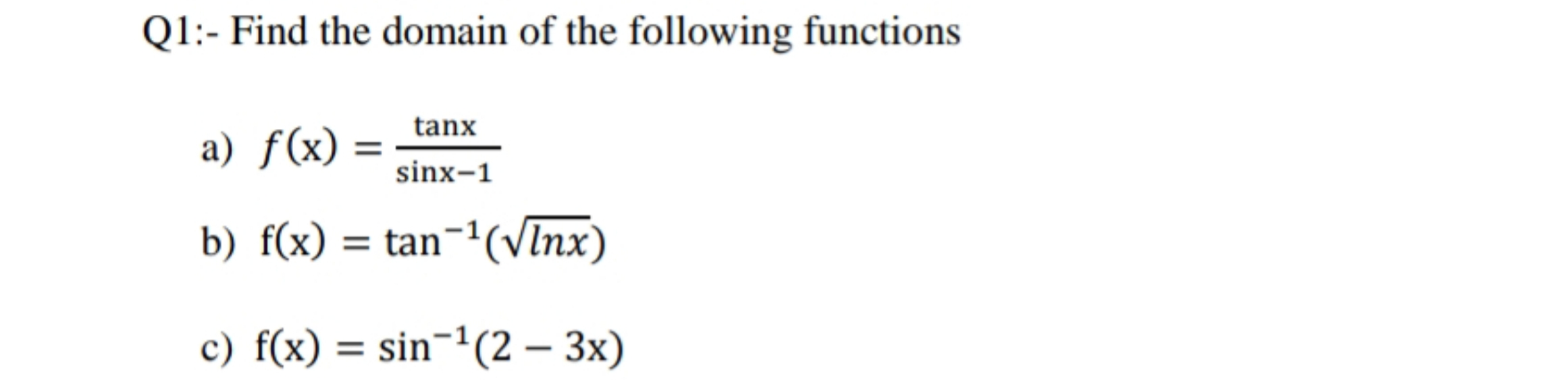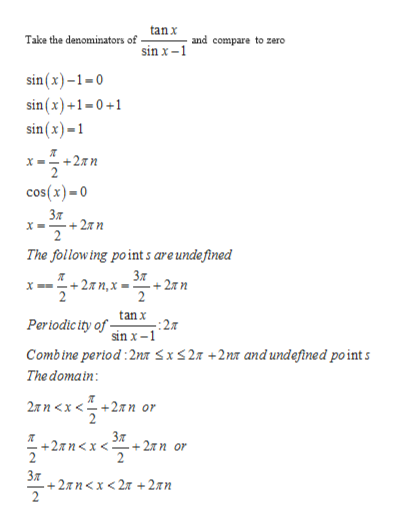# Q1:- Find the domain of the following functionstanxa) f(x)sinx-1b) f(x) tan-(vInx)c) f(x) sin-(2 3x)

Questionhelp_outlineImage TranscriptioncloseQ1:- Find the domain of the following functions tanx a) f(x) sinx-1 b) f(x) tan-(vInx) c) f(x) sin-(2 3x) fullscreen
check_circle

Step 1

Find the domain of the function

Step 2

The Domain of a function is the set of input or argument values for which the function is real and  definedhelp_outlineImage Transcriptionclosetanx Take the denominators of and compare to zero sin x- sin(x)-1-0 sin(x)+1-0+1 sin(x)-1 +2xn cos(x)-0 Зя +27n 22 The follow ing point s are undefined Зя .+ 2лп,х - + 2л п tan x -: 27 sin x-1 Pertodic ity of Combine period:2na sx27 +2n and undefine d point s The domain 2яп <х <+2яп or +2Tnx< 2 + 2ли от 2 Зя +27n < x < 27 + 2an 2 fullscreen
Step 3

The Domain of a function is the set of input or argument values for which the function i...

### Want to see the full answer?

See Solution

#### Want to see this answer and more?

Solutions are written by subject experts who are available 24/7. Questions are typically answered within 1 hour.*

See Solution
*Response times may vary by subject and question.
Tagged in

### Trigonometry